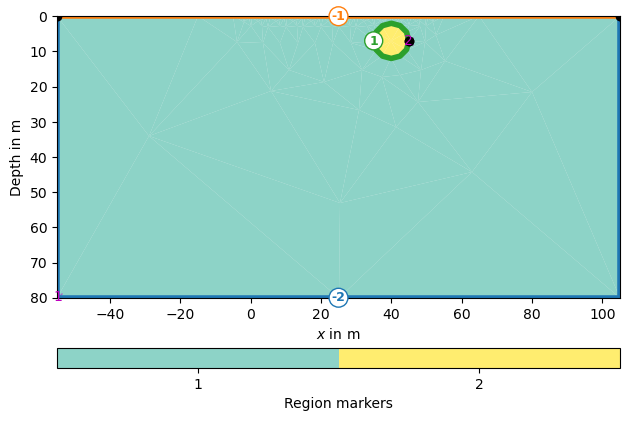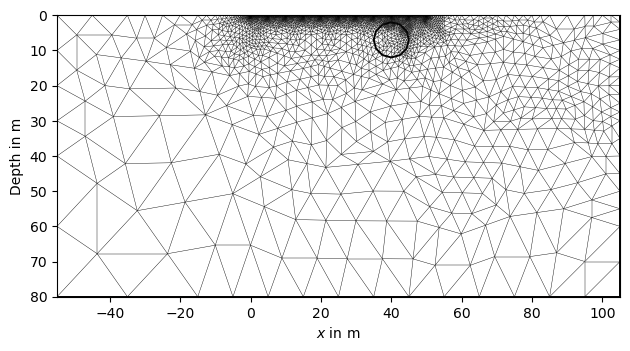# Complex-valued electrical modeling#

In this example, an electrical complex-valued forward modeling is conducted. The use of complex resistivities implies an out-of-phase polarization response of the subsurface, commonly being measured in the frequency domain as complex resistivity (CR), or, if multiple frequencies are measured, also referred to as spectral induced polarization (SIP). Please note that the time-domain induced polarization (TDIP) is a compound signature over a wide range of frequencies.

It is common to parameterize the complex resistivities using magnitude (in $$\Omega$$phi (in mrad), although the pyGIMLi forward operator takes real and imaginary parts.

import numpy as np
import matplotlib.pylab as plt

import pygimli as pg
import pygimli.meshtools as mt
from pygimli.physics import ert


Create a measurement scheme for 51 electrodes with a spacing of 1m

scheme = ert.createData(
elecs=np.linspace(start=0, stop=50, num=51),
schemeName='dd'
)


Mesh generation

world = mt.createWorld(
start=[-55, 0], end=[105, -80], worldMarker=True)

polarizable_anomaly = mt.createCircle(
)

plc = world + polarizable_anomaly

# local refinement of mesh near electrodes
for s in scheme.sensors():
plc.createNode(s + [0.0, -0.2])

mesh_coarse = mt.createMesh(plc, quality=33)
mesh = mesh_coarse.createH2()

# Create a P2-optimized mesh (quadratic shape functions)
mesh = mesh.createP2()

pg.show(plc, marker=True)
pg.show(plc, markers=True)
pg.show(mesh)

•••(<matplotlib.axes._subplots.AxesSubplot object at 0x7ff386618e20>, None)


Prepare the model parameterization We have two markers here: 1: background 2: circle anomaly Parameters must be specified as a complex number, here converted by the utility function pygimli.utils.complex.toComplex().

rhomap = [
[1, pg.utils.complex.toComplex(100, 0 / 1000)],
# Magnitude: 100 ohm m, Phase: -50 mrad
[2, pg.utils.complex.toComplex(100, -50 / 1000)],
]

# For visualization, map the rhomap into the actual mesh, resulting in a rho
# vector with a complex resistivity associated with each mesh cell.
rho = pg.solver.parseArgToArray(rhomap, mesh.cellCount(), mesh)
fig, axes = plt.subplots(2, 2, figsize=(16 / 2.54, 16 / 2.54))
pg.show(mesh, data=np.real(rho), ax=axes[0, 0], label=r"$\rho'$~[$\Omega$m]")
pg.show(mesh, data=np.imag(rho), ax=axes[0, 1], label=r"$\rho''$~[$\Omega$m]")
pg.show(mesh, data=np.abs(rho), ax=axes[1, 0], label=r"$|\rho$|~[$\Omega$m]")
pg.show(
mesh, data=np.arctan2(np.imag(rho), np.real(rho))*1000,
ax=axes[1, 1], label=r"$\phi$ [mrad]"
)
fig.tight_layout()
fig.show()Do the actual forward modeling

data = ert.simulate(
mesh,
res=rhomap,
scheme=scheme,
# noiseAbs=0.0,
# noiseLevel=0.0,
)


Visualize the modeled data Convert magnitude and phase into a complex apparent resistivity

rho_a_complex = data['rhoa'].array() * np.exp(1j * data['phia'].array())

# Please note the apparent negative (resistivity) phases!
fig, axes = plt.subplots(2, 2, figsize=(16 / 2.54, 16 / 2.54))
ert.showERTData(data, vals=data['rhoa'], ax=axes[0, 0])
ert.showERTData(
data, vals=data['phia'] * 1000, label=r'$\phi$ [mrad]', ax=axes[0, 1])

ert.showERTData(
data, vals=np.real(rho_a_complex), ax=axes[1, 0],
label=r"$\rho_a'$~[$\Omega$m]"
)
ert.showERTData(
data, vals=np.imag(rho_a_complex), ax=axes[1, 1],
label=r"$\rho_a''$~[$\Omega$m]"
)
fig.tight_layout()
fig.show()
`Total running time of the script: ( 0 minutes 18.923 seconds)

Gallery generated by Sphinx-Gallery